2021 NBA Most Improved Player of the Year Odds
+1000
10 to 1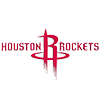Kevin Porter
5.1% implied probability

No changes have been recorded yet.
+1000
10 to 1Michael Porter
5.1% implied probability

+1200
12 to 1Jordan Poole
4.3% implied probability

+1600
16 to 1Jaren Jackson
3.3% implied probability

+1800
18 to 1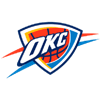Shai Gilgeous-Alexander
3.0% implied probability

+2000
20 to 1OG Anunoby
2.7% implied probability

+2000
20 to 1Collin Sexton
2.7% implied probability

+2000
20 to 1Zion Williamson
2.7% implied probability

No changes have been recorded yet.
+2500
25 to 1Ja Morant
2.2% implied probability

+2500
25 to 1Terance Mann
2.2% implied probability

+3000
30 to 1Darius Garland
1.8% implied probability

+3000
30 to 1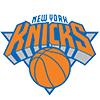Immanuel Quickley
1.8% implied probability

+3000
30 to 1RJ Barrett
1.8% implied probability

No changes have been recorded yet.
+3000
30 to 1Christian Wood
1.8% implied probability

+3000
30 to 1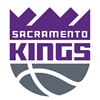De'Aaron Fox
1.8% implied probability

+3500
35 to 1Fred VanVleet
1.6% implied probability

+3500
35 to 1Nickeil Alexander-Walker
1.6% implied probability

No changes have been recorded yet.
+3500
35 to 1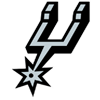Derrick White
1.6% implied probability

+3500
35 to 1Keldon Johnson
1.6% implied probability

No changes have been recorded yet.
+3500
35 to 1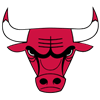Lonzo Ball
1.6% implied probability

No changes have been recorded yet.
+3500
35 to 1Zach LaVine
1.6% implied probability

+3500
35 to 1Jaylen Brown
1.6% implied probability

+3500
35 to 1Tyrese Haliburton
1.6% implied probability

+3500
35 to 1Dejounte Murray
1.6% implied probability

No changes have been recorded yet.
+3500
35 to 1Anthony Edwards
1.6% implied probability

+4000
40 to 1Chris Boucher
1.4% implied probability

+4000
40 to 1LaMelo Ball
1.4% implied probability

No changes have been recorded yet.
+4000
40 to 1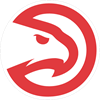John Collins
1.4% implied probability

+4000
40 to 1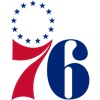Tyrese Maxey
1.4% implied probability

+4500
45 to 1Ben Simmons
1.2% implied probability

+4500
45 to 1Coby White
1.2% implied probability

No changes have been recorded yet.
+4500
45 to 1Domantas Sabonis
1.2% implied probability

+5000
50 to 1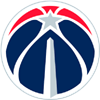Kyle Kuzma
1.1% implied probability

+5000
50 to 1Robert Williams
1.1% implied probability

+5000
50 to 1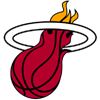Tyler Herro
1.1% implied probability

+5000
50 to 1Mikal Bridges
1.1% implied probability

+5000
50 to 1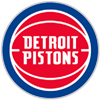Jerami Grant
1.1% implied probability

+5500
55 to 1Saddiq Bey
1.0% implied probability

+6000
60 to 1Bogdan Bogdanovic
0.9% implied probability

+6000
60 to 1Dillon Brooks
0.9% implied probability

+6000
60 to 1Malik Beasley
0.9% implied probability

No changes have been recorded yet.
+6000
60 to 1Deandre Ayton
0.9% implied probability

+6500
65 to 1Darius Bazley
0.9% implied probability

+6500
65 to 1Terry Rozier
0.9% implied probability

+7000
70 to 1Nikola Vucevic
0.8% implied probability

No changes have been recorded yet.
+7000
70 to 1D'Angelo Russell
0.8% implied probability

No changes have been recorded yet.
+7500
75 to 1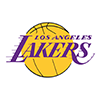Talen Horton-Tucker
0.7% implied probability

+7500
75 to 1Luguentz Dort
0.7% implied probability

No changes have been recorded yet.
+7500
75 to 1Jarrett Allen
0.7% implied probability

+8000
80 to 1Devonte' Graham
0.7% implied probability

No changes have been recorded yet.
+8000
80 to 1Matisse Thybulle
0.7% implied probability

No changes have been recorded yet.
+8000
80 to 1Gary Trent
0.7% implied probability

+8000
80 to 1Kristaps Porzingis
0.7% implied probability

No changes have been recorded yet.
+8000
80 to 1Patrick Williams
0.7% implied probability

No changes have been recorded yet.
+8000
80 to 1Cameron Johnson
0.7% implied probability

No changes have been recorded yet.
+8000
80 to 1Brandon Clarke
0.7% implied probability

+8000
80 to 1Mo Bamba
0.7% implied probability

No changes have been recorded yet.
+8000
80 to 1Markelle Fultz
0.7% implied probability

No changes have been recorded yet.
+8000
80 to 1Wendell Carter
0.7% implied probability

No changes have been recorded yet.
+8000
80 to 1Shake Milton
0.7% implied probability

No changes have been recorded yet.
+8000
80 to 1Bam Adebayo
0.7% implied probability

No changes have been recorded yet.
+8000
80 to 1Mitchell Robinson
0.7% implied probability

+8000
80 to 1CJ McCollum
0.7% implied probability

No changes have been recorded yet.
+8000
80 to 1Rui Hachimura
0.7% implied probability

No changes have been recorded yet.
+9000
90 to 1Marvin Bagley
0.6% implied probability

No changes have been recorded yet.
+9000
90 to 1Seth Curry
0.6% implied probability

No changes have been recorded yet.
+10000
100 to 1Jusuf Nurkic
0.6% implied probability

+10000
100 to 1Miles Bridges
0.6% implied probability

No changes have been recorded yet.
+10000
100 to 1P.J. Washington
0.6% implied probability

No changes have been recorded yet.
+13000
130 to 1Cameron Payne
0.4% implied probability

No changes have been recorded yet.
+13000
130 to 1Kendrick Nunn
0.4% implied probability

No changes have been recorded yet.
+13000
130 to 1Jordan Clarkson
0.4% implied probability

No changes have been recorded yet.
+13000
130 to 1Derrick Jones
0.4% implied probability

No changes have been recorded yet.
+15000
150 to 1Richaun Holmes
0.4% implied probability

No changes have been recorded yet.
+15000
150 to 1Clint Capela
0.4% implied probability

+15000
150 to 1Kyle Anderson
0.4% implied probability

No changes have been recorded yet.
+15000
150 to 1Andrew Wiggins
0.4% implied probability

No changes have been recorded yet.
+15000
150 to 1Malcolm Brogdon
0.4% implied probability

No changes have been recorded yet.